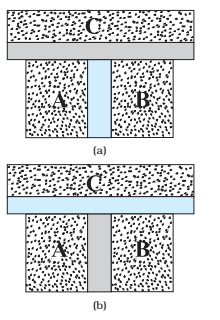Thermodynamics is the branch of physics that deals with the concepts of heat and temperature and the inter-conversion of heat and other forms of energy.

### Thermodynamics versus the kinetic theory of gases:

Thermodynamics is a macroscopic science. It deals with bulk systems and does not go into the molecular constitution of matter. On the other hand, kinetic theory of gases deals with the molecular distribution of velocities in any gas.

### Thermodynamics versus mechanics:

In mechanics, our interest is in the motion of particles or bodies under the action of forces and torques. Thermodynamics is not concerned with the motion of the system as a whole. It is concerned with the internal macroscopic state of the body.

### Thermodynamics system:

An assembly of a very large number of particles having a certain value of pressure, volume and temperature is called a thermodynamics system.

### Thermodynamics variables:

the quantities like pressure (P), volume (V), and temperature (T) which help us to study the behavior of a thermodynamic system are called thermodynamics variables.

### The equation of state:

the mathematical relationship between the pressure, volume and temperature of a thermodynamic system is called its equation of state.

For example, the equation of state for n moles of an ideal gas can be written as,# Thermal Equilibrium

Equilibrium in mechanics means that the net external force and torque on a system are zero.

## Thermal equilibrium:

Two systems are said to be in thermal equilibrium with each other if they have the same temperature.

Consider two gases A and B occupying two different containers. We know experimentally that pressure and volume of a given mass of gas can be chosen to be its two independent variables.

• Let the pressure and volume of the gases be (PA, VA) and (PB, VB) respectively. Suppose first that the two systems are put in proximity but are separated by an adiabatic wall – an insulating wall (can be movable) that does not allow the flow of energy (heat) from one to another.
• The situation is shown schematically in Fig. (a). In this case, it is found that any possible pair of values (PA, VA) will be in equilibrium with any possible pair of values (PB, VB).
• Next, suppose that the adiabatic wall is replaced by a diathermic wall – a conducting wall that allows energy flow (heat) from one to another.
• It is then found that the macroscopic variables of the systems A and B change spontaneously until both the systems attain equilibrium states. After that, there is no change in their states.(a) Systems A and B (two gases) separated by an adiabatic wall – an insulating wall that does not allow flow of heat. (b) The same systems A and B separated by a diathermic wall – a conducting wall that allows heat to flow from one to another. In this case, thermal equilibrium is attained in due course.

• The situation is shown in Fig. (b). The pressure and volume variables of the two gases change to (PB’, VB’) and (PA′, VA′) such that the new states of A and B are in equilibrium with each other.
• There is no more energy flow from one to another. We then say that the system A is in thermal equilibrium with the system B.

# Zeroth law of Thermodynamics

Zeroth law of thermodynamics states that if two system A and B are in thermal equilibrium with a third system C, then the two system A and B are also in thermal equilibrium with each other.Systems A and B are separated by an adiabatic wall, while each is in contact with a third system C via a conducting wall.(b) The adiabatic wall between A and B is replaced by a conducting wall, while C is insulated from A and B by an adiabatic wall.